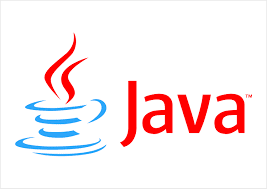# java - Beginner Guide to Epoch Time with examples

### Epoch time IntroductionEpoch time is a number of seconds that already passed from 1 January 1970 UTC time. Every Day the total number of seconds is 24* 60*60 seconds. Epoch time return the long number between the current time and 1 January 1970 time

Epoch time is also called Unix time. Every programming language provides API for handling Unix time. Java also provides Date API for manipulation of Epoch Time.

#### Epcoh/unix time Examples

Following are the frequently used examples for manipulation of Epoch time in java.

#### How to calculate Epoch time or Unix time in java?

Epoch time is a number of seconds that already passed since 1970 January 1 UTC. Prior to java8, Used System class to get Epoch time,

``````long epochTimeinSeconds = System.currentTimeMillis() / 1000L;
System.out.println(epochTimeinSeconds);

``````

With java8, Date and Time API is improved and introduced java.time package for dealing with date, time and zones over legacy classes.\ Instant is a class of point in a time zone.

``````long epochTimewithJava8 = Instant.now().getEpochSecond();
System.out.println(epochTimewithJava8);

``````

Both return the same output

``````1535948933

``````

#### How to convert Date and time to Epoch time seconds?

java.time.Instant class provides toEpochMilli() method to convert the date with time into epoch milliseconds.

``````long epochTimewithJava81= Instant.now().toEpochMilli(); //Long = 1450879900184
System.out.println(epochTimewithJava81);

``````

#### Convert epoch time java.time.LocalDateTime

LocalDateTime represents Date and time zone information, epoch time is in long seconds. For this, we need to have System Default Timezone retrieved and the Instant object is required to convert to it

``````
long epochTimewithJava8 = System.currentTimeMillis();
System.out.println(epochTimewithJava8);
Instant instant = Instant.ofEpochMilli(epochTimewithJava8);
LocalDateTime localDateTime = instant.atZone(ZoneId.systemDefault()).toLocalDateTime();
System.out.println(localDateTime);

``````

The output of the above java program is

`````` 1535949786956
2018-09-03T10:13:06.956
``````

#### Convert epoch time to LocalDate

LocalDate represents date only. To convert, Instant object creation is required with default timezone

``````long epochTimewithJava8 = System.currentTimeMillis();
System.out.println(epochTimewithJava8);
Instant instant = Instant.ofEpochMilli(epochTimewithJava8);
LocalDate localDate = instant.atZone(ZoneId.systemDefault()).toLocalDate();
System.out.println(localDate);

``````

Output is

``````1535950017609
2018-09-03

``````

#### How to Convert String Date to Epoch Time.

String date is first converted to Date using SimpleDateFormat. Date format class accepts a formatted string and returns Date object by calling parse()method

``````String str="2018-09-03T04:51:00+05:0";
DateFormat parser = new SimpleDateFormat("yyyy-MM-dd'T'HH:mm:ssX");
Date date=null;
try {
date = parser.parse(str);
} catch (ParseException e) {
// TODO Auto-generated catch block
e.printStackTrace();
}
System.out.println(date.getTime()/1000l);

``````

Output is

``````1535932260

``````
Similar Posts
Subscribe
You'll get a notification every time a post gets published here.

Related posts